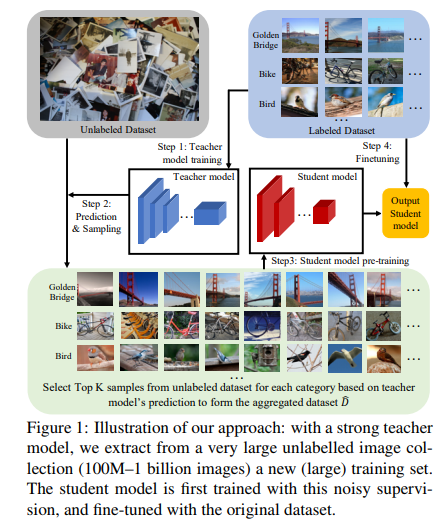Last Commit
May. 15, 2019
Created
May. 3, 2019

# Implementing Billion-scale semi-supervised learning for image classification using Pytorch

## Network Architecture• Step 1:
• We train on the labeled data to get an initial teacher model
• Step 2:
• For each class/label, we use the predictions of this teacher model to rank the unlabeled images and pick top-K images to construct a new training data
• Step 3:
• We use this data to train a student model, which typically differs from the teacher model: hence we can target to reduce the complexity at test time
• Step 4:
• finally, pre-trained student model is fine-tuned on the initial labeled data to circumvent potential labeling errors.

## Usage

• Step 1:
• If there is a pretrained weight of the teacher network, go to step 2.
• If you do not have pretrained weights, run the following command to train the teacher network.
``````python main.py
``````
• Step 2:
• Sampling unlabeled data through a pretrained teacher network.
``````python make_sample_data.py
``````
• Step 3:
• Students learn the student network using the data sampled in Step 2.
``````python student_train.py
``````
• Step 4:
• Finally, fine-tuning the CIFAR-100 data using the student network trained using unlabeled data in Step 3.
``````python main.py --student-network True
``````

### Unlabeled Data

• Image crawler

## Experiments

• In the paper, K=16k, P=10, Dataset=ImageNet, Unlabeled Data: 1,000,000,000 images.
• However, we do not have many GPUs, so we are training at CIFAR-100.
• Ours) K=1000, P=10, Dataset=CIFAR-100, Unlabeled Data: About 150,000 images.
Datasets Model Accuracy Epoch Training Time
CIFAR-100 ResNet-50 76.36% 91 3h 31m
CIFAR-100 ResNet-50, Semi-Supervisied learning(WORK IN PROCESS)
• For CIFAR-100 data, the image size is too small, so the result is not good when the unlabeled data is reduced to (32, 32).
• We will solve this problem !!

## Requirements

• tqdm==4.31.1
• torch==1.0.1
• opencv version: 4.1.0